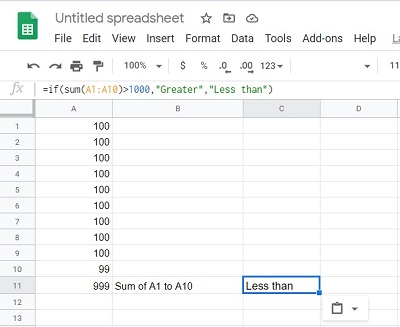# How to Use IF THEN Statements in Google Sheets

Even without using functions, Google Sheets is still a remarkable tool for business or study. Knowing how to use functions like IF and THEN, however, expands its usefulness almost exponentially.In this article, we’ll explain everything you need to know about the IF THEN statements in Google Sheets, and show examples of how to use them.

The IF THEN Statement operates like a simple logic question. The function checks whether a statement is true or not then returns a value depending on the answer. You can look at the function as ‘IF this is TRUE, THEN do this, otherwise do something else’. Keeping this in mind when you create IF THEN statements will simplify the coding into chunks of information that you can easily understand.

The syntax is pretty simple until you get in to nested IF statements. That is, an IF statement within an IF statement. Even then, the only complication isn’t that it’s hard to create a formula, but that it’s easy to misplace values, especially for longer IF THEN statements. If you don’t lose sight of the fact that you’re just making a long logic question though, it makes it easier to find any potential errors.## The Syntax

The Google Sheets IF THEN Function can be used by using the following syntax: =IF(Logical Expression, value-if-true,value-if-false) where:

‘=’ indicates to Google Sheets that you’re using a function.

‘IF’ indicates that the values in the parenthesis will be tested to be true or false.

‘Logical Expression’ is the condition that is to be tested.

‘value-if-true’ is what will be returned if the logical expression is true.

=value-if-false’ is what will be returned if the logical expression is false.

For example, if you want to test whether cell A1 is greater than cell A2, then display the value GREATER if it is, and LESS THAN if it’s not, then you’ll write the formula as =IF(A1>A2,”GREATER”,”LESS THAN”).

You can also insert other functions into the statement, such as the SUM function. For example, if you want to see if the sum of cells A1 to A10, is over 1000, then you can write the formula as =IF(SUM(A1:A10)>1000,”GREATER”,”LESS THAN”).## Nested IF THEN Statements

Nested IF THEN statements operate on the same logic as a normal IF THEN statement. It checks if a logical expression is true or not, then returns a value if it is. This time, however, instead of returning a value immediately if the expression is false, it checks if another logical expression is true or not. This process repeats until you get to the end of a formula. A simple example would be the determination of a grade. If your grade is 90 and above you get an A, if you’re 70 to 89, you get a B, 50 to 69 will get you a C, 35 to 49 will get you a D, and any less will get you an F.

If you were to write this into a nested IF THEN statement then you’d get ‘=IF(B1>89,”A”,if(B1>69,”B”,if(B1>49,”C”,if(B1>34,”D”,”F”))))’. Google Sheets will check each condition one by one and if it finds a condition that’s true it will stop. The Nested IF function will try to find a true condition. If it does, it will no longer check the other conditions.

This is important to remember if you want different results for particular values. In the example above, if you had written the formula to check if the grade was higher than 35 first before checking any other value, then any grade higher than 35, whether it’s 36 or 99, will stop there. It will no longer check to see if it meets the other conditions, and will return the value-if-true that you entered. Keep this in mind when making your formula.## Using the AND / OR Statement with IF THEN Statements

If you want to check the truth value of more than one logical expression before returning a result, you can utilize the AND / OR function on your IF THEN Statement. The AND function returns True if all the parameters you enter are true, and the OR function will return true if at least one of the parameters is true.

The Syntax of AND Statements go by =AND(Logical Expression1,Logical Expression2, Logical Expression3…), while OR shares the similar syntax =OR(Logical Expression1, logical expression2…). As using the SUM function, you omit the ‘=’ to avoid encountering errors.

For example, you wish to evaluate the values of two cells, A1 and A2 to see if their values are equal to or greater than 100. The formula for an IF statement would then be =IF(AND(A1>99,A2>99),”True”,”False”). This will only return true if both cells are 100 or more, and return false even if only one is less than 100. Using OR will return true if at least one is greater than 100.If you wish to further evaluate more cells, you can either expand upon the AND / OR function, or nest further IF THEN Statements. As long as you can simplify a condition to a true or false question, the uses for this are limited only by your imagination.

## Simple but Extremely Versatile

The IF THEN function for Google Sheets makes it easier for users to automatically assign results for different values. Its limits are only bound on how well you can construct a logical condition. The utility of this simple, but extremely versatile function can’t be over emphasized.

Do you know of other ways to utilize the IF THEN Statement in Google Sheets? Share your thoughts in the comments section below.

Disclaimer: Some pages on this site may include an affiliate link. This does not effect our editorial in any way.

Todays Highlights